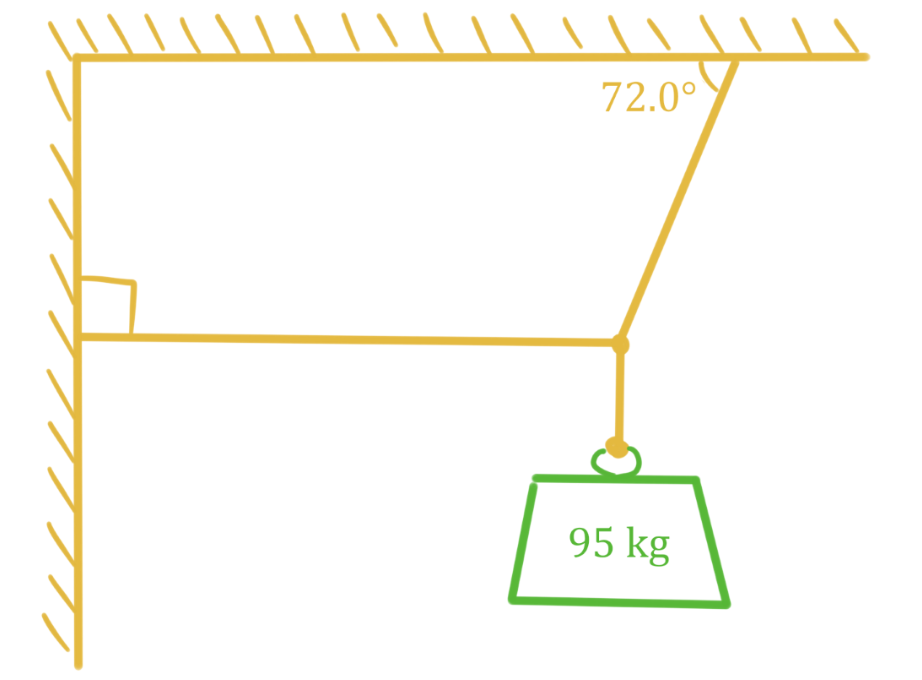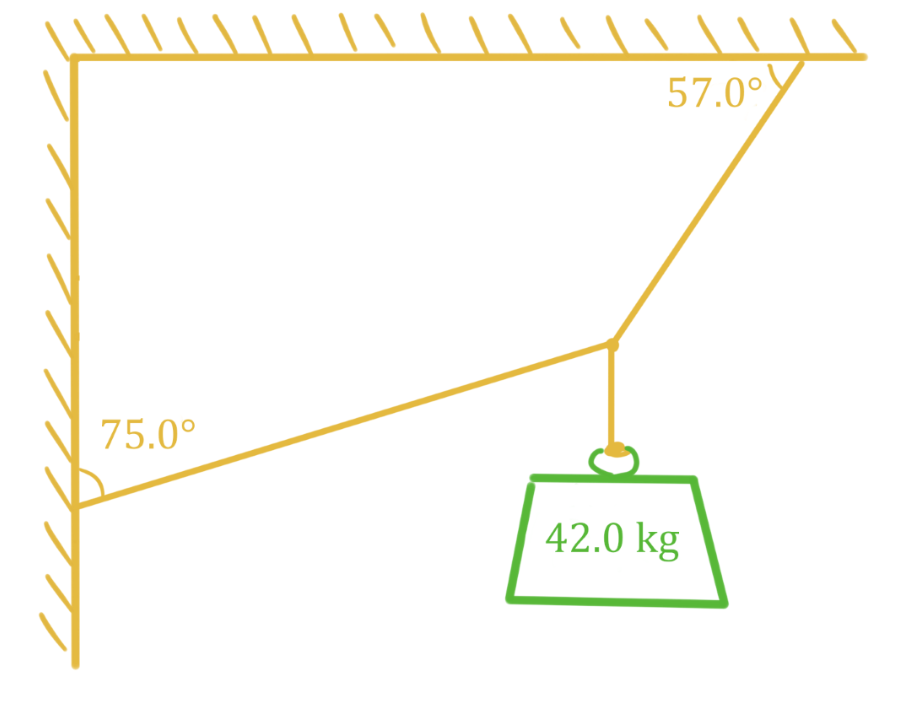# Translational equilibrium

### Translational equilibrium

#### Lessons

In this lesson, we will learn:

• Meaning of translational equilibrium
• Solving problems involving translational equilibrium

Notes:

• An object can undergo translational motion (motion that changes its position) and rotational motion (motion that changes its angle).
• An object is in translational equilibrium if the velocity of its translational motion is constant. An object that is not moving or an object that is moving in a straight line at a constant velocity would be considered in translation equilibrium. To be in translational equilibrium, the net force acting on the object must be zero (recall Newton's first law: if no unbalanced forces act on an object, it does not accelerate).
Conditions for Translational Equilibrium

$\Sigma F = 0 N$

or equivalently:

$\Sigma F_{x} = 0 N$ and $\Sigma F_{y} = 0 N$

$\Sigma F:$ sum of all forces, in newtons (N)

$\Sigma F_{x}:$ sum of all force components in x direction, in newtons (N)

$\Sigma F_{y}:$ sum of all force components in y direction, in newtons (N)

• 1.
Translational equilibrium of hanging masses
a)

A 95.0 kg object is suspended by two cables attached to the ceiling and wall as shown. What is the tension in cable attached to the wall?b)

A 42.0 kg object is suspended by two cables attached to the ceiling and wall as shown. What is the tension in cable attached to the wall?